Published

# Golf Ball Dispenser!

Golf Ball dispenser for the laziest of golfers who don't want to even push a golf ball into position with their club!

IntermediateFull instructions provided20 hours509## Things used in this project

### Hardware components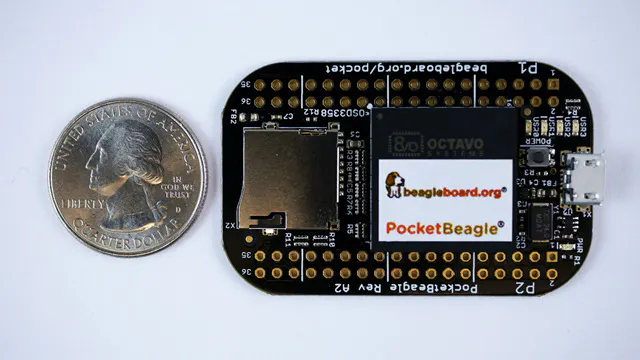BeagleBoard.org PocketBeagle
×1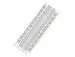×1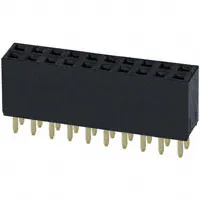Female Header 20 Position 2 Row (0.1")
×2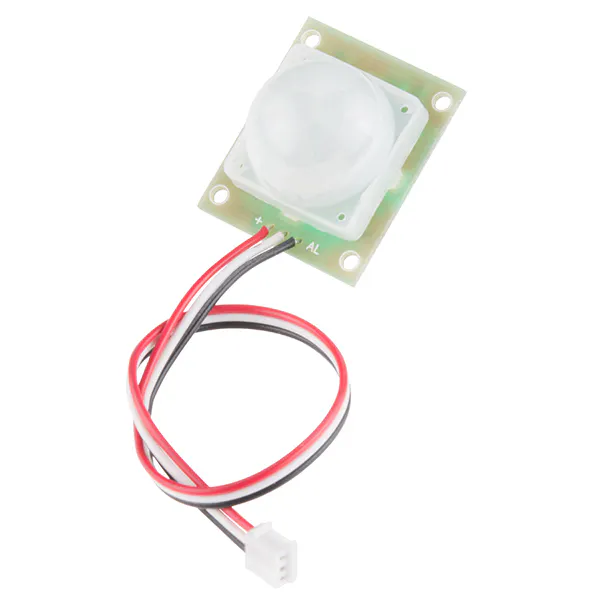PIR Motion Sensor (generic)
×1
 Adafruit Standard servo - TowerPro SG-5010 - 5010
×1
 male/male jumper wires
×1
 WIFI USB dongle
×1
 breakout board with USB port
×1
×1

## Schematics

### System Block Diagram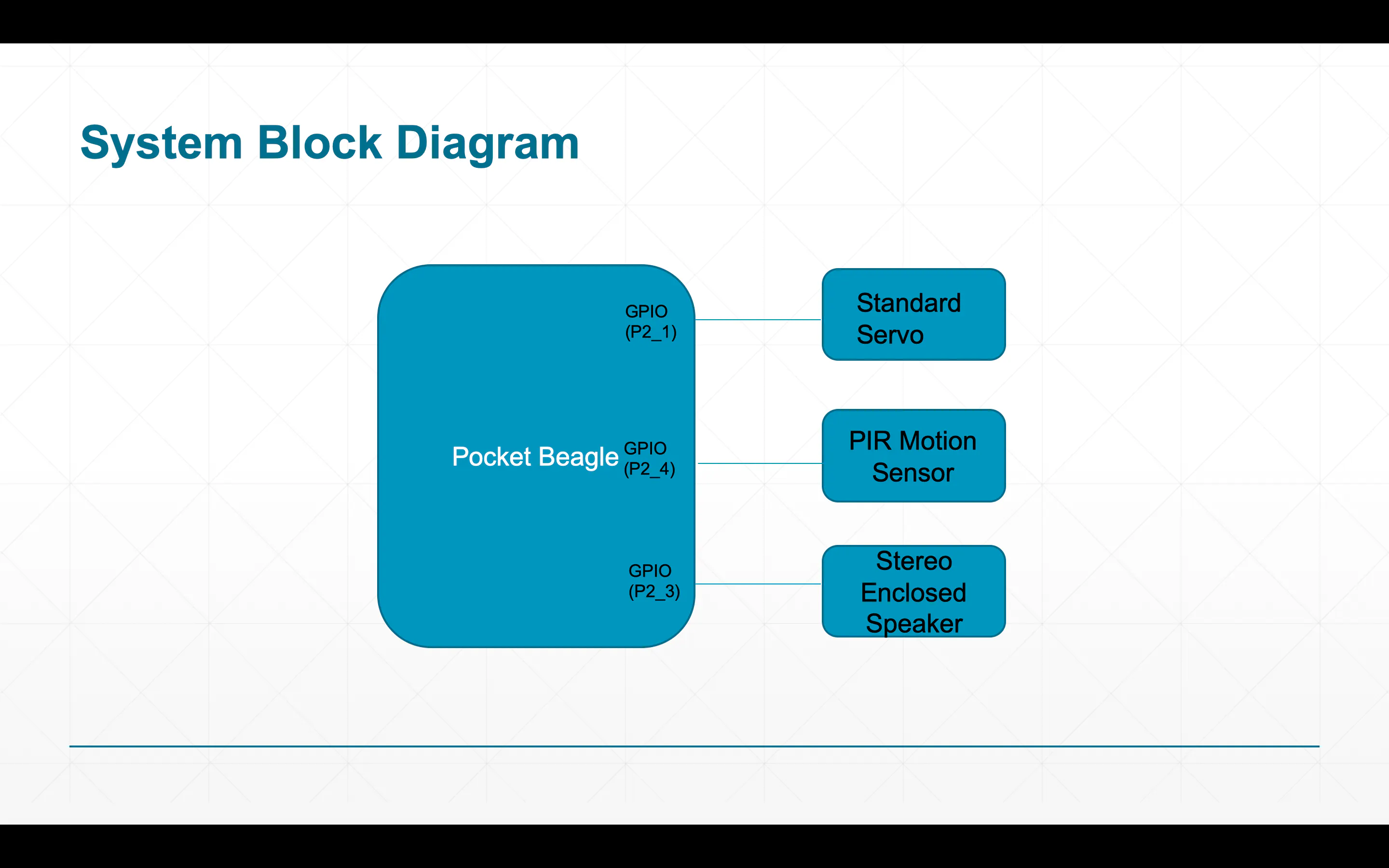### Power Block Diagram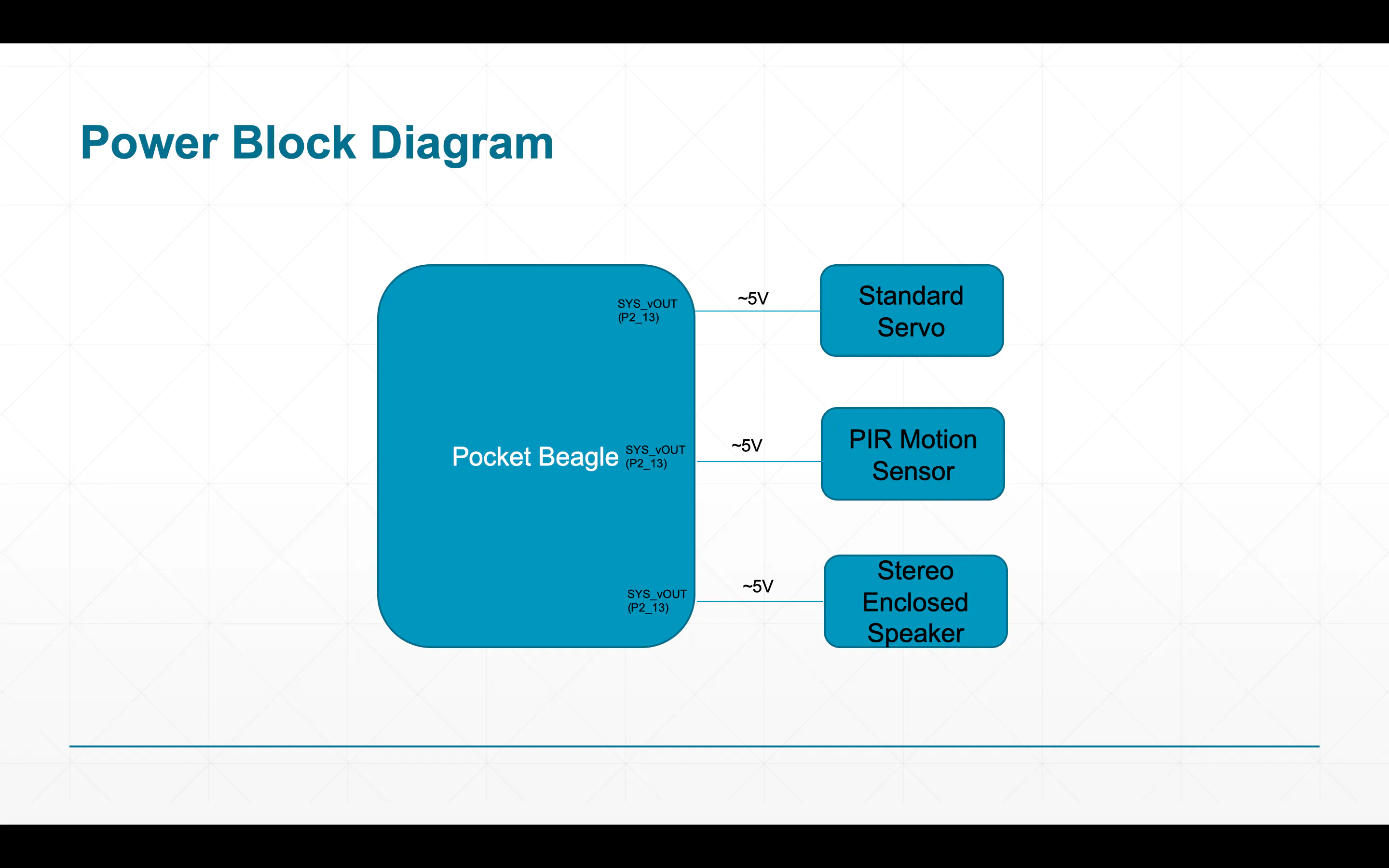## Code

### Golf Ball Dispenser Code

Python
This is the dispenser python code for my project
```"""
--------------------------------------------------------------------------
Golf Ball Dispenser
--------------------------------------------------------------------------

Redistribution and use in source and binary forms, with or without
modification, are permitted provided that the following conditions are met:

1. Redistributions of source code must retain the above copyright notice, this
list of conditions and the following disclaimer.

2. Redistributions in binary form must reproduce the above copyright notice,
this list of conditions and the following disclaimer in the documentation
and/or other materials provided with the distribution.

3. Neither the name of the copyright holder nor the names of its contributors
may be used to endorse or promote products derived from this software without
specific prior written permission.

THIS SOFTWARE IS PROVIDED BY THE COPYRIGHT HOLDERS AND CONTRIBUTORS "AS IS"
AND ANY EXPRESS OR IMPLIED WARRANTIES, INCLUDING, BUT NOT LIMITED TO, THE
IMPLIED WARRANTIES OF MERCHANTABILITY AND FITNESS FOR A PARTICULAR PURPOSE ARE
DISCLAIMED. IN NO EVENT SHALL THE COPYRIGHT HOLDER OR CONTRIBUTORS BE LIABLE
FOR ANY DIRECT, INDIRECT, INCIDENTAL, SPECIAL, EXEMPLARY, OR CONSEQUENTIAL
DAMAGES (INCLUDING, BUT NOT LIMITED TO, PROCUREMENT OF SUBSTITUTE GOODS OR
SERVICES; LOSS OF USE, DATA, OR PROFITS; OR BUSINESS INTERRUPTION) HOWEVER
CAUSED AND ON ANY THEORY OF LIABILITY, WHETHER IN CONTRACT, STRICT LIABILITY,
OR TORT (INCLUDING NEGLIGENCE OR OTHERWISE) ARISING IN ANY WAY OUT OF THE USE
OF THIS SOFTWARE, EVEN IF ADVISED OF THE POSSIBILITY OF SUCH DAMAGE.
--------------------------------------------------------------------------
This program uses a PIR motion sensor to detect movement. Upon detecting movement,
it gives pir_value a value of 1, and if the value is 1, then the servo rotates a
specified amount so that it allows a golf ball to be dispensed, and a little jing
is played right before it dispenses.
Requirements: Dispense a ball and play a jingle upon motion.

"""

import time

#Imports needed BBIO libraries for the servo and notes to work

#Note Library
#--------------------------------------------------------
NOTE_B0  = 31
NOTE_C1  = 33
NOTE_CS1 = 35
NOTE_D1  = 37
NOTE_DS1 = 39
NOTE_E1  = 41
NOTE_F1  = 44
NOTE_FS1 = 46
NOTE_G1  = 49
NOTE_GS1 = 52
NOTE_A1  = 55
NOTE_AS1 = 58
NOTE_B1  = 62
NOTE_C2  = 65
NOTE_CS2 = 69
NOTE_D2  = 73
NOTE_DS2 = 78
NOTE_E2  = 82
NOTE_F2  = 87
NOTE_FS2 = 93
NOTE_G2  = 98
NOTE_GS2 = 104
NOTE_A2  = 110
NOTE_AS2 = 117
NOTE_B2  = 123
NOTE_C3  = 131
NOTE_CS3 = 139
NOTE_D3  = 147
NOTE_DS3 = 156
NOTE_E3  = 165
NOTE_F3  = 175
NOTE_FS3 = 185
NOTE_G3  = 196
NOTE_GS3 = 208
NOTE_A3  = 220
NOTE_AS3 = 233
NOTE_B3  = 247
NOTE_C4  = 262
NOTE_CS4 = 277
NOTE_D4  = 294
NOTE_DS4 = 311
NOTE_E4  = 330
NOTE_F4  = 349
NOTE_FS4 = 370
NOTE_G4  = 392
NOTE_GS4 = 415
NOTE_A4  = 440
NOTE_AS4 = 466
NOTE_B4  = 494
NOTE_C5  = 523
NOTE_CS5 = 554
NOTE_D5  = 587
NOTE_DS5 = 622
NOTE_E5  = 659
NOTE_F5  = 698
NOTE_FS5 = 740
NOTE_G5  = 784
NOTE_GS5 = 831
NOTE_A5  = 880
NOTE_AS5 = 932
NOTE_B5  = 988
NOTE_C6  = 1047
NOTE_CS6 = 1109
NOTE_D6  = 1175
NOTE_DS6 = 1245
NOTE_E6  = 1319
NOTE_F6  = 1397
NOTE_FS6 = 1480
NOTE_G6  = 1568
NOTE_GS6 = 1661
NOTE_A6  = 1760
NOTE_AS6 = 1865
NOTE_B6  = 1976
NOTE_C7  = 2093
NOTE_CS7 = 2217
NOTE_D7  = 2349
NOTE_DS7 = 2489
NOTE_E7  = 2637
NOTE_F7  = 2794
NOTE_FS7 = 2960
NOTE_G7  = 3136
NOTE_GS7 = 3322
NOTE_A7  = 3520
NOTE_AS7 = 3729
NOTE_B7  = 3951
NOTE_C8  = 4186
NOTE_CS8 = 4435
NOTE_D8  = 4699
NOTE_DS8 = 4978

# Stating Variables
# ------------------------------------------------------------------------
servo_pin = "P2_1"
pir_value = 0
PIR_pin = "P2_4" # Pin number connected to PIR sensor output wire.
speaker_pin = "P2_3"

# functions
# ------------------------------------------------------------------------

def jingle_song():
play_note(NOTE_F5, 0.35)
play_note(NOTE_C5, 0.20)
play_note(NOTE_C5, 0.20)
play_note(NOTE_D5, 0.20)
play_note(NOTE_C5, 0.35)
play_note(NOTE_E5, 0.20)
play_note(NOTE_F5, 0.20)
PWM.stop(speaker_pin)
#Stops the sounds
PWM.cleanup()
#Cleans up the speaker so that it doesnt keep making the jingle
def dispense_ball():
#function for the servo moving a certain amount to dispense the ball
PWM.start(servo_pin, (100), 20.0)
PWM.set_duty_cycle(servo_pin, 0.5)

time.sleep(1.0)
#Waits a second for it to turn back to original position
PWM.start(servo_pin, (100), 20.0)
PWM.set_duty_cycle(servo_pin, 3.5)

time.sleep(1.0)

PWM.stop(servo_pin)
PWM.cleanup()

# end def
def play_note(Note, Length):
#Defines the function for playing a certain note in the jingle_song() def
PWM.start(speaker_pin, 50, Note)
time.sleep(Length)
#end def

# Main script
# ------------------------------------------------------------------------

#Digital input for PIR sensor
direction = GPIO.setup(PIR_pin, GPIO.IN)

#Gets output from GPIO port:
pir = GPIO.input(PIR_pin)

old_value = pir_value

while True:
pir_value = GPIO.input(PIR_pin)
print("pir_value = {0}".format(pir_value))

if pir_value:
# PIR is detecting movement, pir_value would equal to 1
if not old_value:

jingle_song()
#Plays jingle
dispense_ball()
#Dispenses ball (Moves servo)
print('Motion detected')
else:
if old_value:
print('No motion detected')
old_value = pir_value
time.sleep(2.0)
#Since this is a while loop, it will keep going until stopped by hitting
#control c on the keyboard.

```

### run_Dispenser2.sh

Plain text
This shell script navigates the software to the right directory, configures pins, and runs the main script.
```#!/bin/bash
"""
--------------------------------------------------------------------------
Golf Ball Dispenser Dispenser Run Shell Script
--------------------------------------------------------------------------

Redistribution and use in source and binary forms, with or without
modification, are permitted provided that the following conditions are met:

1. Redistributions of source code must retain the above copyright notice, this
list of conditions and the following disclaimer.

2. Redistributions in binary form must reproduce the above copyright notice,
this list of conditions and the following disclaimer in the documentation
and/or other materials provided with the distribution.

3. Neither the name of the copyright holder nor the names of its contributors
may be used to endorse or promote products derived from this software without
specific prior written permission.

THIS SOFTWARE IS PROVIDED BY THE COPYRIGHT HOLDERS AND CONTRIBUTORS "AS IS"
AND ANY EXPRESS OR IMPLIED WARRANTIES, INCLUDING, BUT NOT LIMITED TO, THE
IMPLIED WARRANTIES OF MERCHANTABILITY AND FITNESS FOR A PARTICULAR PURPOSE ARE
DISCLAIMED. IN NO EVENT SHALL THE COPYRIGHT HOLDER OR CONTRIBUTORS BE LIABLE
FOR ANY DIRECT, INDIRECT, INCIDENTAL, SPECIAL, EXEMPLARY, OR CONSEQUENTIAL
DAMAGES (INCLUDING, BUT NOT LIMITED TO, PROCUREMENT OF SUBSTITUTE GOODS OR
SERVICES; LOSS OF USE, DATA, OR PROFITS; OR BUSINESS INTERRUPTION) HOWEVER
CAUSED AND ON ANY THEORY OF LIABILITY, WHETHER IN CONTRACT, STRICT LIABILITY,
OR TORT (INCLUDING NEGLIGENCE OR OTHERWISE) ARISING IN ANY WAY OUT OF THE USE
OF THIS SOFTWARE, EVEN IF ADVISED OF THE POSSIBILITY OF SUCH DAMAGE.
--------------------------------------------------------------------------
Moves to proper directory where the dispenser code is located, and runs Dispenser2.py

"""
cd /var/lib/cloud9/ENGI301/project_01
#Navigates to proper directory

config-pin P2_1 pwm
config-pin P2_3 pwm
config-pin P2_4 pwm

#Configures pins
python3 Dispense2.py
#Runs the program
```

## Credits

### Luis Perales

1 project • 0 followers